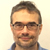# U-value| Posted in Energy Efficiency and Durability on

I have two units. Both are 50m2. The first has 10m x 3m (width x height)of exposed facade comprised of 50% glass at R-3 and 50% wall rated at R-30. The second has only 5m of exposed facade but it is entirely 100% glass at R-3. Which one will be more energy efficient?

I’ve asked a few people and have had different takes. Some believe that because the R-value will be higher in the first unit it will be more energy efficient. However, since the second has less exposed facade, I have also had some believe that it is more energy efficient.

Any experts care to weigh in???

thanks!## Join the leading community of building science experts

### Replies

1.GBA Editor
| | #1

Yvan,
In neither case is the phrase "energy efficient" relevant. This has nothing to do with efficiency.

What you are interested in calculating is the rate of heat flow.

The heat loss formula for determining transmission losses through floors, roofs, and walls is:
Q = A • U • ΔT
In other words, the rate of heat flow through a building assembly (in Btu/h) is equal to the area of the assembly (in ft²) times the U-factor (in Btu/ft² • hr • F°) of the assembly times the ΔT (in F°). The ΔT (delta-T) is the difference in temperature between the interior and the exterior of the building.

Since I think in English (non-metric) units, I'll convert your area measurements to square feet.
30 square meters = 333 square feet
50% of 30 square meters = 15 square meters = 161.5 square feet

Case 1, assuming a ΔT of 50 F°:
Q = [161.5 * 0.33 * 50] + [161.5 * 0.033 *50] = 2,931 Btu/h

Case 2, assuming a ΔT of 50 F°:
Q = 161.5 * 0.33 * 50 = 2,664 Btu/h

So the rate of heat flow through the narrower facade is less than the rate of heat flow through the wider facade.

I chose a delta-T of 50 F° to illustrate the method. The delta-T was arbitrary. Regardless of the delta-T, the ratio of the heat loss between these two facades will stay the same; regardless of the outdoor temperature, the narrower facade will always lose heat at a slower rate than the wider facade.

2.Expert Member
| | #2

A house or room with 15 square meters of window can have air conditioning requirements that would cause the cooling bills to exceed the heating bills, even in cooler climates.

The U-factor is just one important consideration, the SHGC (solar heat gain coefficient), and the direction the window is facing can be just as important, or even MORE important when there is a LOT of window. Fifteen square meters of "sunset view" west facing glass with an SHGC of 0.5 or greater can easily double or triple the peak cooling load of a house, turning it into a solar oven on every sunny afternoon/evening, any time of year.

3.| | #3

It's so close that it may come down to which one is better air sealed (where delta-T matters). Or solar gain.

• |
• |
• |
• |